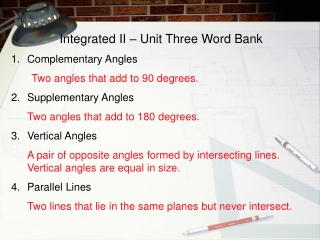DownloadDownload PresentationIntegrated II – Unit Three Word Bank Complementary Angles Two angles that add to 90 degrees.

# Integrated II – Unit Three Word Bank Complementary Angles Two angles that add to 90 degrees.

Télécharger la présentation## Integrated II – Unit Three Word Bank Complementary Angles Two angles that add to 90 degrees.

- - - - - - - - - - - - - - - - - - - - - - - - - - - E N D - - - - - - - - - - - - - - - - - - - - - - - - - - -
##### Presentation Transcript

1. Integrated II – Unit Three Word Bank Complementary Angles Two angles that add to 90 degrees. Supplementary Angles Two angles that add to 180 degrees. Vertical Angles A pair of opposite angles formed by intersecting lines. Vertical angles are equal in size. Parallel Lines Two lines that lie in the same planes but never intersect.

2. Integrated II – Unit Three Word Bank • Parallelogram • A quadrilateral with two pairs of parallel sides • Rectangle • A parallelogram with four right angles • Rhombus • A parallelogram with four congruent sides • Square • A parallelogram with four right angles and four congruent sides

3. Integrated II – Unit Three Word Bank • Trapezoid • A quadrilateral with exactly one pair of parallel sides • Quadrilateral • A four-sided polygon • Polygon • A closed shape made up of line segments • Regular Polygon • A polygon that has all congruent sides and all congruent angles.

4. Integrated II – Unit Three Word Bank • Convex Polygon • A polygon where the extension of no sides enters the polygon • Concave Polygon • A polygon where the extension of one or more sides enters the polygon • Pentagon • A five-sided polygon • Hexagon • A six-sided polygon

5. Integrated II – Unit Three Word Bank • Heptagon (Septagon) • A seven-sided polygon • 18. Octagon • An eight-sided polygon • 19. Nonagon • A nine-sided polygon • Decagon • A ten-sided polygon

6. Integrated II – Unit Three Word Bank • Acute Triangle • A triangle with three acute angles • Obtuse Triangle • A triangle with one obtuse angle • Right Triangle A triangle with one right angle • Translation • A movement of an image to a new location with out changing its’ relative position, aka a slide

7. Integrated II – Unit Three Word Bank 25. Equilateral Triangle A triangle with three equal side lengths 26. Isosceles Triangle A triangle with two equal side lengths 27. Scalene Triangle A triangle with no equal side lengths Rotation Movement of an image around a fixed point

8. Integrated II – Unit Three Word Bank 29. Reflection Movement of an image over a fixed line creating a mirror image 30. Pre-Image A shape before a transformation 31. Image A shape after a transformation